# Kinetics Activity

-Introduction

-Background Step 1

-Background Step 2

-Step 1: Making a kinetic trace

-Step 2a: Getting information from a kinetic trace

-Step 2b: Determining the pseudo rate constant k'

-Step 3: Determine the effect of bleach on the reaction

-Step 4: Converting absorbance to concentration

Kinetics Studies of the Bleaching of Food Dyes >> Background Step 1

 < Previous | Next >

# Kinetics Studies of the Bleaching of Food Dyes

## Background Step 1 - Obtaining a kinetic trace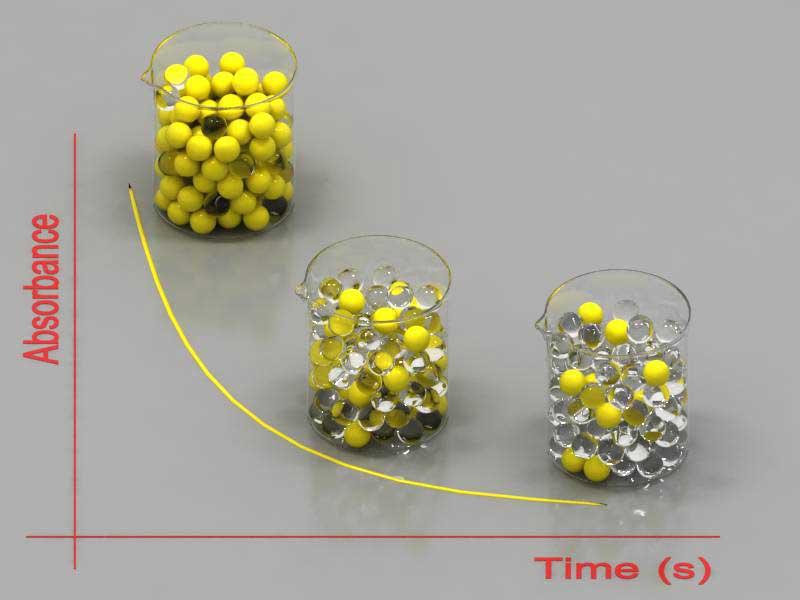When a dye solution reacts with bleach, its colour fades (or more precisely its absorbance decreases) due to the decrease in dye's concentration. A plot of absorbance versus time (shown to the right) is a "kinetic trace" of the reaction. Note that the colour fades because as dye is bleached (symbolized as yellow spheres becoming clear spheres), the number of dye molecules (yellow spheres) decreases with time and the colour fades.

## Introduction: The Rate Law

The notion of a fast versus slow reaction is fairly intuitive: explosion of a balloon filled with hydrogen is a fast reaction; rusting of metal is a slow reaction. But how do we quantify the rate of the reaction of dye with bleach? The rate of a reaction is defined as the rate of change in concentration of a reactant or a product. Since the dye is the only coloured species in solution, we can monitor the decrease in its concentration with time:where the exponents m and n indicate the order of the reaction with respect to each reactant, and k is the rate constant. The goal of this experiment is to determine the order of the reaction with respect to the dye and to the bleach (m and n) and the value of k. To determine those, we need to leave the world of mathematical formalisms and enter the laboratory.

## Taking advantage of bleach being present in large excess

In all of the experiments we will be carrying out, the concentration of bleach is much larger than that of the dye. We can use this while interpreting the experiment. As an example, suppose our initial concentrations are:

[dye] = 1.0 mM and [bleach] = 180 mM.

and we get these kinetic traces for dye and bleach: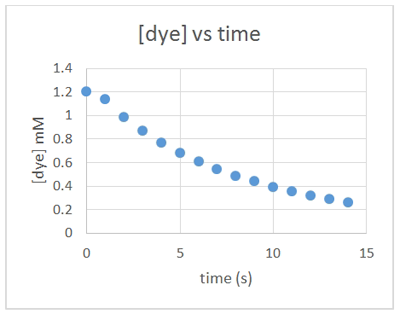Plot of [dye] versus time [y axis 0 to 1.2 mM]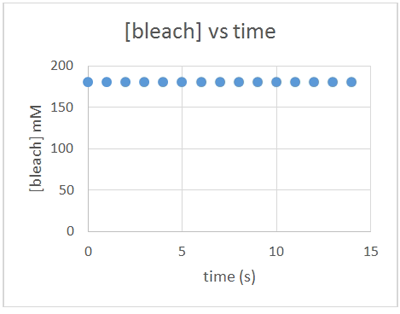Plot of [bleach] versus time [y axis 0 to 200 mM]

As the reaction proceeds, [dye] drops from 1.0 to nearly 0 mM, but [bleach] essentially stays at 180 mM. In other words, [bleach] stays constant throughout the experiment. In the rate law: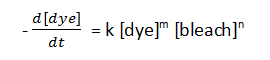[bleach] is constant and so can be combined with the rate constant k: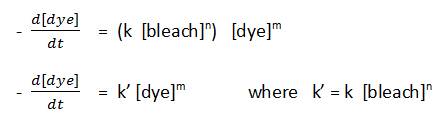k' is a constant that combines the rate constant with the concentration of bleach. We call k' the pseudo-rate constant.

Please apply this information as you answer the following question:

 < Previous | Next >
 Contact Us Last Updated: Thursday, March 10th, 2022 @ 05:15:38 am